# Solution assignment 01 Root equations

### Assignment 1

Solve: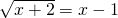### Solution

The following conditions have to be satisfied (because of the square roots):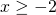and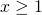so:Squaring both sides yields the equation: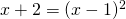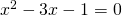This quadratic equation has the following solutions (by applying the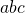-formula):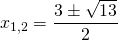or: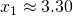of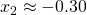The first equation satisfies the condition above, the second does not.

The solution: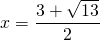satisfies the original equation.